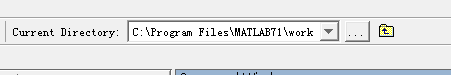• 第一步： 新建m文件，并写函数: function f = fun(x) f = x+1 第二步：保存到work目录下，不然不能用 第三步：在command窗口输入函数名，并...（在这里函数名笔者定义的是fun，则在命令行中调用也应该是fun（x） ...
第一步： 新建m文件，并写函数:
function f = fun(x)
f = x+1
第二步：保存到work目录下，不然不能用第三步：在command窗口输入函数名，并传入参数。（在这里函数名笔者定义的是fun，则在命令行中调用也应该是fun（x）
注:如果还是无法调用，请把.M文件的名字跟函数名相同


展开全文• @matlab调用函数如何知道被调用函数的其他变量的值 在使用MATLAB过程中，我们难免会遇到函数调用函数的情况，外面的主程序中的变量我们可以在工作区看到，他们的值的变化我们可以清楚的知道，但是主程序中调用的...
@matlab中调用函数，如何知道被调用函数的其他变量的值
在使用MATLAB过程中，我们难免会遇到函数调用函数的情况，外面的主程序中的变量我们可以在工作区看到，他们的值的变化我们可以清楚的知道，但是主程序中调用的一些函数的变量的值我们却很难知道，此时，我们可以采取如下方法：
（1）将主程序中函数末尾的分号去掉，再次运行程序，在命令行窗口我们就可以看到函数中自变量的值；
然而，被调用函数的程序中的一些变量、常量的值我们如何知道呢？
（2）在被调用函数的某处直接加入一段程序即可，这段程序就是你这个想知道的变量的名字，不加分号，回到主程序，运行，即可在命令行窗口看到这个变量的变化的值。


展开全文变量
• K_matrix.m”的matlab函数文件'包含以下代码：function K = calculate_K_matrix(A, B, n)K = place(A, B, eigs(A)*n)end我可以这样从matlab调用它：^{pr2}$但当我尝试使用matlab引擎API从python调用函数时，... 我有一个名为“calculate_K_matrix.m”的matlab函数文件'包含以下代码：function K = calculate_K_matrix(A, B, n)K = place(A, B, eigs(A)*n)end我可以这样从matlab中调用它：^{pr2}$但当我尝试使用matlab引擎API从python调用此函数时，如下所示：import matlabimport matlab.engineeng = matlab.engine.start_matlab()A = matlab.double([[0, 1],[-100, -5]])B = matlab.double([,])n = 1.1                  double(param initializer=None, param size=None, param is_complex=False)n_matlab = matlab.double([n])eng.addpath(r'/home/ash/Dropbox/SimulationNotebooks/Control')K = eng.calculate_K_matrix(A, B, n_matlab)然后我得到以下错误：In : run test.pyAttempt to execute SCRIPT calculate_K_matrix as a function:/home/ash/Dropbox/SimulationNotebooks/Control/calculate_K_matrix.m---------------------------------------------------------------------------MatlabExecutionError                      Traceback (most recent call last)/home/ash/Dropbox/SimulationNotebooks/Control/test.py in ()1011 eng.addpath(r'/home/ash/Dropbox/SimulationNotebooks/Control')---> 12 K = eng.calculate_K_matrix(A, B, n_matlab)/home/ash/anaconda2/envs/python3/lib/python3.5/site-packages/matlab/engine/matlabengine.py in __call__(self, *args, **kwargs)76         else:77             return FutureResult(self._engine(), future, nargs, _stdout,---> 78                                 _stderr, feval=True).result()7980     def __validate_engine(self):/home/ash/anaconda2/envs/python3/lib/python3.5/site-packages/matlab/engine/futureresult.py in result(self, timeout)66                 raise TypeError(pythonengine.getMessage('TimeoutCannotBeNegative'))67---> 68         return self.__future.result(timeout)6970     def cancel(self):/home/ash/anaconda2/envs/python3/lib/python3.5/site-packages/matlab/engine/fevalfuture.py in result(self, timeout)80                 raise TimeoutError(pythonengine.getMessage('MatlabFunctionTimeout'))81---> 82             self._result = pythonengine.getFEvalResult(self._future,self._nargout, None, out=self._out, err=self._err)83             self._retrieved = True84             return self._resultMatlabExecutionError: Attempt to execute SCRIPT calculate_K_matrix as a function:/home/ash/Dropbox/SimulationNotebooks/Control/calculate_K_matrix.m我如何解决这个问题？在
展开全文• matlab如何查看特定函数在何处被调用 当我们在学习一个包含很多子函数的matlab程序时，某些子函数不知道在何处调用，我们可以通过使用查找命令，快捷键为ctrl+F； 1.以查找GetNurbsSpline函数为例，弹出查找文件...
matlab如何查看特定函数在何处被调用
当我们在学习一个包含很多子函数的matlab程序时，某些子函数不知道在何处调用，我们可以通过使用查找文件命令，它位于主页菜单栏左侧；
1.以查找GetNurbsSpline函数为例，弹出查找文件对话框后；
2.在查找包含以下文本的文件处输入所要查找的函数名GetNurbsSpline，就可以在右边的图框中看到在当前工作文件夹的所有m文件中出现该函数的位置。展开全文• 我们在使用matlab进行图像处理的过程中，经常会用到的一个工具就是折线图！不同于直方图，但性质类似！所以今天我们来讲讲这个非常好用的Plot函数 话不多说，上代码 %%获取视频文件 obj = VideoReader('test2_6....
• 在Java中采用Matlab JA Builder可以实现调用m文件，采用这样的方式，可在...那如何使用Matlab直接调用Java的方式？错误的方法：网上有些说将jar、class放在Matlab的Search路径中。查看了下Matlab的说明，Search...
• 这里写自定义目录标题欢迎使用Markdown编辑器新的改变功能快捷键合理的创建标题，有助于目录的生成如何改变文本的样式插入链接与图片如何插入一段漂亮的代码片生成一个适合你的列表创建一个表格设定内容居中、居左、...
• 一、在 MATLAB 中的窗函数,十分简单： （1）矩形窗（Rectangle Window） 调用格式：w=boxcar(n)，根据长度 n 产生一个矩形窗 w。 （2）三角窗（Triangular Window） 调用格式：w=triang(n)，根据长度...
• 使用MATLAB过程中，我们难免会遇到函数调用函数的情况，外面的主函数中的变量我们可以在工作区看到，他们的值的变化我们可以清楚的知道，但是主程序中调用的一些函数的变量的值我们却很难知道，此时，我们可以采取...
• 我要在matlab使用caffe的函数，但是我不知道应该调用那些函数，具体的顺序是什么，我要实现的是用我自己的数据集去训练一个网络，并且测试...我想要一个详细的调用Caffe函数的代码，或者调用函数的流程（写出函数名）caffe
• 最近做实验需要使用matlab调用opencv的函数，然后就找了下如何使用matlab调用c++程序并设置依赖库。其实使用matlab调用opencv的程序就是在matlab调用c++程序时设置依赖库，因此，该方法同样适用于matlab调用其他的...
• 如何将实验结果在matlab中可视化呢，下面使用java语言编程，调用matlab中的函数：本人安装的是Matlab7.11.0 (R2010a)和 Eclipse 4.2 ；1)首先设置环境变量，运行tomcat，Eclipse等都需要依靠环境变量JAVA_HOME，Path...
• 如何将实验结果在matlab中可视化呢，下面使用java语言编程，调用matlab中的函数： 本人安装的是Matlab7.11.0 (R2010a)和 Eclipse 4.2 ； 1)首先设置环境变量，运行tomcat，Eclipse等都需要依靠环境变量JAVA_HOME，...
• 但是MATLAB中带有实现fft功能的原始程序吗？如何调用
• ## matlab如何在文中定义函数

万次阅读 多人点赞 2017-01-04 14:12:54
matlab如何在文中定义函数 通常我们多是将函数单独在编写在单个的m文件中，再于主程序中调用。但是，有时候觉得程序太小，直接在程序末尾编写函数，又会收到“此上下文中不允许函数定义”的错误提示。那么，matlab...
• 如何使用Matlab调用笔记本摄像头 - Matlab计算机视觉与机器认知学习笔记 - 001如何使用Matlab调用笔记本摄像头1.函数 imaqhwinfo 的介绍及使用2.创建视频输入对象3.预览视频流及关闭4.获取图像、视频数据4.1. 获取...计算机视觉
• 您可以像在MATLAB中那样直接使用结果。参数解析功能用于从系统命令提示符或shell调用python脚本，而不是从pythonshell调用python脚本。因此，有一个类似myscript.py的脚本文件：def metrics(minval, maxval):""...
• 第一步配置环境； 要安装引擎 API，请在操作...或者，使用在非默认位置安装用于 Python 的 MATLAB 引擎 API中所述的非默认选项之一。 在 Windows 系统中 管理员cmd输入： cd "matlabroot\extern\engines\py...
• 接触了MATLAB的许多操作，在这里做下关于调用笔记本摄像头的笔记。 首先需要了解imaqhwinfo函数，在命令行输入imaqhwinfo就可以看到自己笔记本相关适配器和MATLAB版本的信息.但并不能看到摄像头的信息。 >>...
• 欢迎使用Markdown编辑器写博客笔记是记录通过java如何调用matlab函数： 1.在命令行中输入deploytool 2.点击上图中标有红框的部分 3.选择javapackage，依次填写name，classname 在package的过程中可能会出现...
• smulink可以很方便的搭建系统...Matlabfunction函数框如下： 1、首先定义全局变量globala; 通过EditData 新建变量a,并且属性Scope选择Data store memory 2、通过simulink View-》ModelExplore——》BaseWo...
• Matlab是一个强大的数学计算/仿真工具，其内置了很多实用的现成的函数，而且我们经常也自己定义很多m函数。但在很多情况下，我们不得不使用VC编程。那么，如何在VC中利用matlab的资源呢？ 在这里我简要的以一个简单...
• 这个小代码示例演示了如何通过调用Python脚本在MATLAB使用 。 依存关系 此代码示例需要位于/home/user/python/env文件夹中的Python虚拟环境。 pip install numpy pip install openslide-python 不要忘记根据您的...
• 在进行算法验证的时候，Matlab无疑是一把利器，如果将用C写的代码，在用Matlab实现一遍，确实很...如何在C中方便地使用Matlab传递过来的变量？   由于Matlab中常使用double型变量，而C中最常使用的是int型变量，当
• 设计思路：首先在eclipse中使用java调用Matlab函数，再在Oracle存储过程中将java类映射其中。 （1）首先讲解如何利用eclipse调用matlab函数，以及个人遇到的一些问题和问题的解决思路。 准备工作：安装jdk，jdk的...oracle eclipse java jdk
• 使用matlab编辑了一个计算卷积的函数。 输入的变量如下 function R = reliability( range,q1,q2,b1,b2,k1,k2,l1,l2 ) 计算之后，返回一个数组。 用matlab编译成.dll文件，在VS使用c#调用时遇到以下问题![图片说明]...c#
• ## vs2013调用matlab函数

千次阅读 2015-05-05 19:05:58
添加matlab环境，这个网上都有，然后使用 Engine *ep; if(!(ep = engOpen(""))) { cout ; }打开engine，下面主要介绍如何将参数传进去（主要是c++中的类）： mxCreateStructMatrix函数：mxArray *mxCreateSt
• matlabcontrol-4.0.0.jar" HelloWorld将打开一个MATLAB会话，并显示输出： hello world hello from MYFUNC您也可以将文件夹添加到路径一次，然后使用SAVEPATH将其保留。这样你就不必每次都这样做。
• 昨天的那一篇讲的几个函数，不知道你们理解的如何，是否懂得怎么去使用了，如果还没懂，一定要再多看几遍，并且去在软件上进行实操，今天的话，将要介绍一下函数的嵌套，不过在正式讲嵌套之前，先对主函数和子函数做......

# matlab如何使用调用函数matlab 订阅